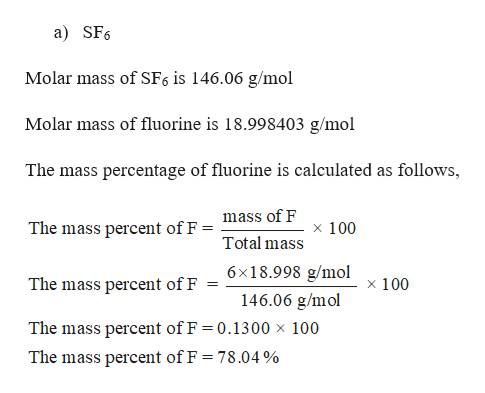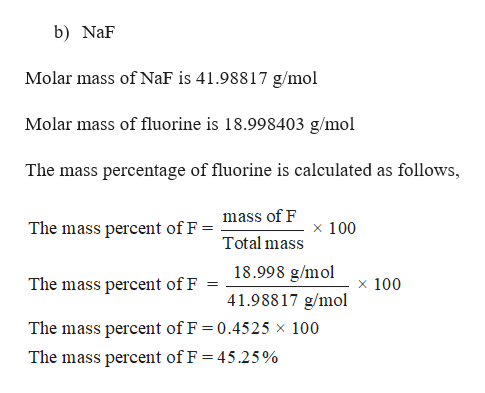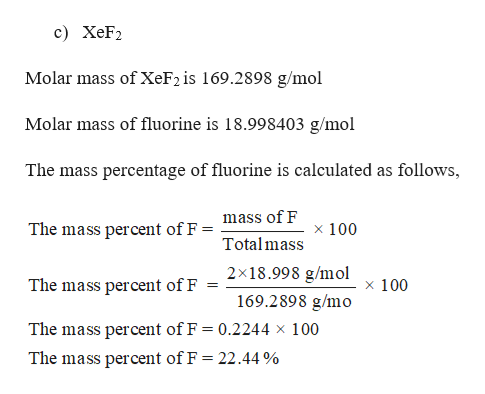# Which one of the following has the smallest mass percent of F in the compound?A) SF₆B) NaFC) XeF₂D) KrF₄

Question
24 views
Which one of the following has the smallest mass percent of F in the compound?
A) SF₆
B) NaF
C) XeF₂
D) KrF₄

check_circle

Step 1

The mass percentage of fluorine is 78.04 %.help_outlineImage Transcriptionclosea) SF6 Molar mass of SF6 is 146.06 g/mol Molar mass of fluorine is 18.998403 g/mol The mass percentage of fluorine is calculated as follows, mass of F The mass percent of F = x 100 Total mass 6x18.998 g/mol The mass percent of F x 100 146.06 g/mol The mass percent of F 0.1300 x 100 The mass percent of F = 78.04 % fullscreen
Step 2

The mass percentage of fluorine is 45.25 %.help_outlineImage Transcriptioncloseb) NaF Molar mass of NaF is 41.98817 g/mol Molar mass of fluorine is 18.998403 g/mol The mass percentage of fluorine is calculated as follows, mass of F The mass percent of F = x 100 Total mass 18.998 g/mol The mass percent of F x 100 41.98817 g/mol The mass percent of F 0.4525 x 100 The mass percent of F = 45.25% fullscreen
Step 3

The mass percentage of fl...help_outlineImage Transcriptionclosec) XeF2 Molar mass of XeF2 is 169.2898 g/mol Molar mass of fluorine is 18.998403 g/mol The mass percentage of fluorine is calculated as follows, mass of F The mass percent of F x 100 Total mass 2x18.998 g/mol 169.2898 g/mo x 100 The mass percent of F The mass percent of F = 0.2244 x 100 The mass percent of F = 22.44 % fullscreen

### Want to see the full answer?

See Solution

#### Want to see this answer and more?

Solutions are written by subject experts who are available 24/7. Questions are typically answered within 1 hour.*

See Solution
*Response times may vary by subject and question.
Tagged in

### General Chemistry